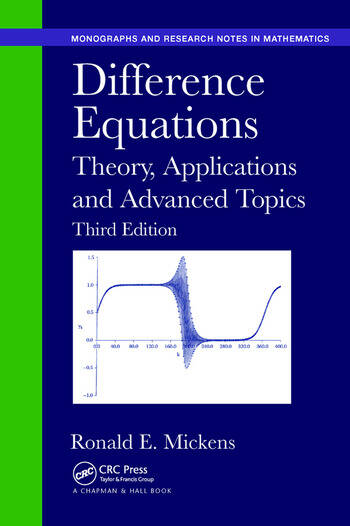# Difference Equations: Theory, Applications and Advanced Topics, Third Edition

## 3rd Edition

Ronald E. Mickens

Chapman and Hall/CRC
Published April 9, 2018
Reference - 555 Pages
ISBN 9781138894235 - CAT# K32876
Series: Chapman & Hall/CRC Monographs and Research Notes in Mathematics

For Instructors Request Inspection Copy

USD\$72.95

FREE Standard Shipping!

## Preview

### Summary

Difference Equations: Theory, Applications and Advanced Topics, Third Edition provides a broad introduction to the mathematics of difference equations and some of their applications. Many worked examples illustrate how to calculate both exact and approximate solutions to special classes of difference equations. Along with adding several advanced topics, this edition continues to cover general, linear, first-, second-, and n-th order difference equations; nonlinear equations that may be reduced to linear equations; and partial difference equations.

New to the Third Edition

• New chapter on special topics, including discrete Cauchy–Euler equations; gamma, beta, and digamma functions; Lambert W-function; Euler polynomials; functional equations; and exact discretizations of differential equations
• New chapter on the application of difference equations to complex problems arising in the mathematical modeling of phenomena in engineering and the natural and social sciences
• Additional problems in all chapters
• Expanded bibliography to include recently published texts related to the subject of difference equations

Suitable for self-study or as the main text for courses on difference equations, this book helps readers understand the fundamental concepts and procedures of difference equations. It uses an informal presentation style, avoiding the minutia of detailed proofs and formal explanations.

#### Instructors

We provide complimentary e-inspection copies of primary textbooks to instructors considering our books for course adoption.